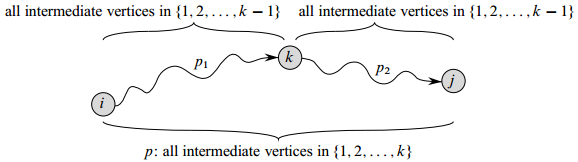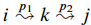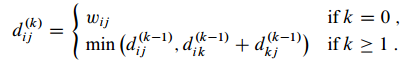# All-Pairs Shortest-Paths (Floyd-Warshall Algorithm)

Updated:

⚠️ This post was created when I was in high school and is no longer maintained.

## 1. Basics

The Floyd-Warshall algorithm is a dynamic-programming formulation to solve the all-pairs shortest-path problem on a directed graph $G = (V, E)$. Same as the Bellman-Ford algorithm, negative-weight edges may be present in $G$ but still no negative-weight cycle is permitted.

### 1.1 Similar relaxation process:

Unlike Dijkstra’s algorithm where each edge only gets to be relaxed once, this algorithm presents a relaxation procedure which I think quite resembles that of the Bellman-Ford algorithm in a sense that they both have about $\mid V \mid$ (outer) iterations in the relaxation process.

### 1.2 Different structures of the shortest path

The Floyd-Warshall algorithm considers all the possible intermediate vertices, the “pit stop” mentioned previously, of a simple path.

The algorithm is based upon the following observation. Under the assumption that the vertices of $G$ are $V = { 1, 2, …, n }$, we consider a subset ${ 1, 2, …, k }$ of the vertices for some $k$. For any pair of the vertices $i, j ∈ V$, consider all paths from $i$ to $j$ whose intermediate vertices are all drawn from ${ 1, 2, …, k }$, and let $p$ be a minimum-weight path from among them.

Note that we assume path $p$ is simple.

The Floyd-Warshall algorithm exploits a relationship between path $p$ and shortest paths from i to j with all intermediate vertices in the set ${1, 2, …, k}$.

This relationship depends on whether or not $k$ is an intermediate vertex of path $p$.• [Relationship 1] If $k$ is not an intermediate vertex of path $p$, then all intermediate vertices of path $p$ are in the set ${1, 2,…, k - 1}$. Thus, a shortest path from vertex $i$ to vertex $j$ with all intermediate vertices in the set ${1, 2,…, k - 1}$ is also a shortest path from $i$ to $j$ with all intermediate vertices in the set ${1, 2,…, k}$.

• [Relationship 2] If $k$ is an intermediate vertex of path $p$, then we decompose $p$ intoas shown above. By the Lemma 24.1 (in CLRS) , $p_1$ is a shortest path from $i$ to $k$ with all intermediate vertices in the set ${1, 2,…, k}$. Because vertex $k$ is not an intermediate vertex of path $p_1$, we see that $p_1$ is a shortest path from i to k with all intermediate vertices in the set ${1, 2,…, k - 1}$. Similarly, $p_2$ is a shortest path from vertex $k$ to vertex $j$ with all intermediate vertices in the set ${1, 2,…, k - 1}$.

In a nutshell, the two different relationships represent different decision-making processes. In respect of the first relationship, we do not take $k$ into consideration, since it’s not the optimal solution, whilst for the second one we do.

## 2. Recursive mindset

To take the two relationships elaborated in 1.2 together, we can now construct a recursive solution for this problem as follows:Tips:

• For $k == 0$, we do the “house-keeping” work, initialization.
• The formula of the case where $k>=1$ clearly illustrates the decision-making process based on different relationships that we mentioned in 1.2.
• when $k == 0$, a path from vertex $i$ to vertex $j$ with no intermediate vertex numbered higher than 0 has no intermediate vertices whatsoever.

## 3. Bottom-up DP approach (a better solution)

An implementation of Floyd-Warshall where the floydwarshall() function creates a matrix M that populates the matrix with shortest path information for each vertex:

class Edge:
def __init__(self, start, end, weight):
self.start = start
self.end = end
self.weight = weight

class Graph:
def __init__(self):
# The adjacency matrix that holds the graph data.
self.vertex_count = 0

if vertex != self.vertex_count:
return "Don't skip a vertex"
self.vertex_count += 1

return "Starting vertex not in graph"
return "Ending vertex not in graph"
if start == end:
return "Cannot have same start and end vertex"
edge = Edge(start, end, weight)

def does_edge_exist(self, start, end):
if edge.start == start and edge.end == end:
return (True, edge)
return (False, None)

def floydwarshall(self):
import sys
for x in range(len(M)):
for y in range(len(M)):
if x == y:
M[x][y] = 0
exists, edge = self.does_edge_exist(x, y)
if exists:
M[x][y] = edge.weight
for k in range(len(M)):
for i in range(len(M)):
for j in range(len(M)):
new_distance = M[i][k] + M[k][j]
if new_distance < M[i][j]:
M[i][j] = new_distance
return M


Tags: